Related Articles
Systematic Sampling in Pandas
• Difficulty Level : Medium
• Last Updated : 24 Feb, 2021

Sampling is the method where one can take subset (Sample) from the given data and will investigate on the sample without investigating each individual thing of data. For instance, suppose in a College, someone wants to check the average height of Students who are Studying in the college. One way is to Collect data of all student and will calculate that but this task is very time-consuming. Thus, sampling is used. So, the solution is that during the Recess, randomly choose Students from Canteen and measure their height and will calculate the average height from that Subset of Student’s.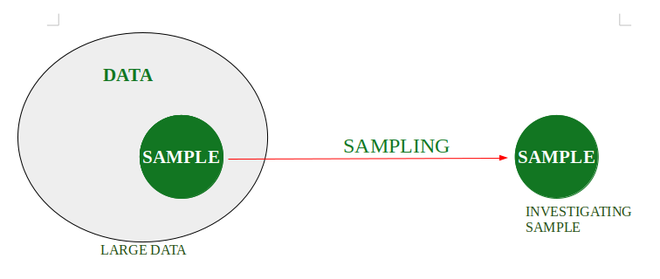Types Of Sampling :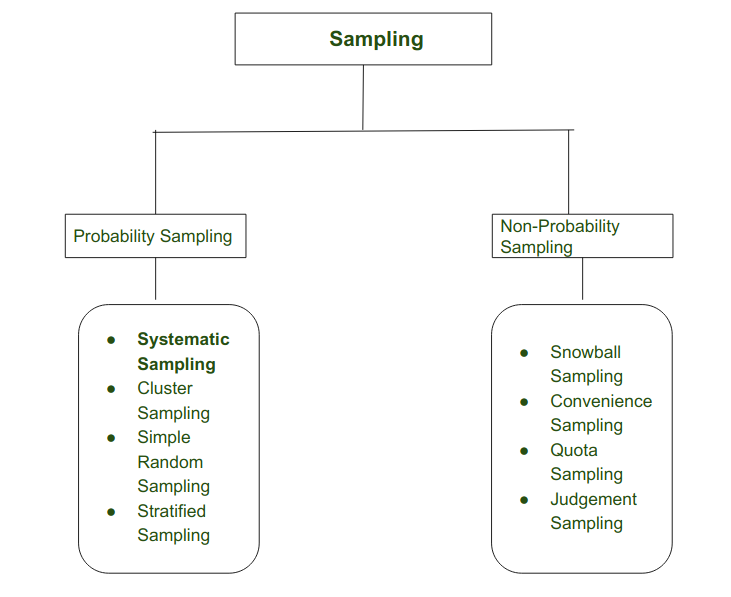Sampling

## Systematic Sampling

Systematic Sampling is defined as the type of Probability Sampling where a researcher can research on a targeted data from large set of data. Targeted data is chosen by selecting random starting point and from that after certain interval next element is chosen for sample. In this a small subset (sample) is extracted from large data.

Suppose that size of Data is D and N will be the Size of Sample that we want to Select. So according to Systematic Sampling :

Interval = (D/N)

Suppose (D/N) = J

So when we choose first random element E from Data , the next element for Sample would be (E+J)

Example : Total Size of Data  = 50 (1 to 50)

We want elements in  Sample = 5

Interval = 50/5 = 10 .

It means in a sample we want gapping of 10 elements Systematically.

Suppose i randomly choose element first Sample Element  = 5

So next would be 5+10 = 15

15+10= 25

25+ 10 =35

35+10  = 45

So,

Sample = { 5,15,25,35,45 }

Diagrammatically,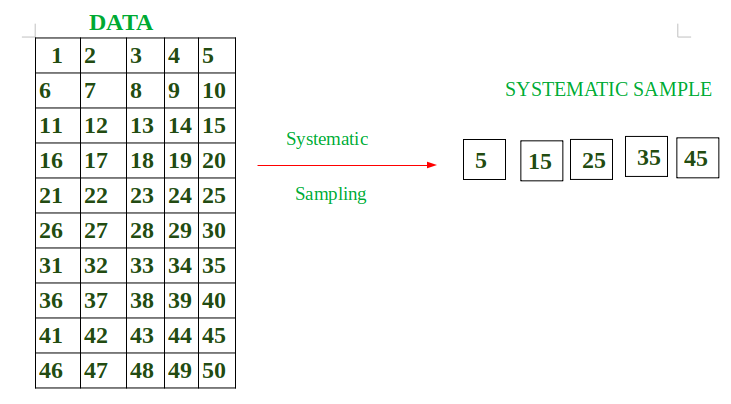Approach:

• Take Data.
• Extract Systematic Sample from large Data.
• Print the Average of Sample Data.

Program:

## Python3

 `# Import in order to use inbuilt functions``import` `numpy as np``import` `pandas as pd`` ` `# Define total number of students``number_of_students ``=` `15`` ` `# Create data dictionary``data ``=` `{``'Id'``: np.arange(``1``, number_of_students``+``1``).tolist(),``        ``'height'``: [``159``, ``171``, ``158``, ``162``, ``162``, ``177``, ``160``, ``175``,``                   ``168``, ``171``, ``178``, ``178``, ``173``, ``177``, ``164``]}`` ` `# Transform dictionary into a data frame``df ``=` `pd.DataFrame(data)`` ` `display(df)`` ` `# Define systematic sampling function``def` `systematic_sampling(df, step):`` ` `    ``indexes ``=` `np.arange(``0``, ``len``(df), step``=``step)``    ``systematic_sample ``=` `df.iloc[indexes]``    ``return` `systematic_sample`` ` ` ` `# Obtain a systematic sample and save it in a new variable``systematic_sample ``=` `systematic_sampling(df, ``3``)`` ` `# View sampled data frame``display(systematic_sample)`

Output: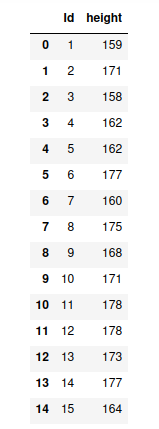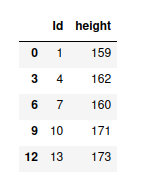Example: Print Average of Sample Data

## Python3

 `# Import in order to use inbuilt functions``import` `numpy as np``import` `pandas as pd`` ` `# Define total number of students``number_of_students ``=` `15`` ` `# Create data dictionary``data ``=` `{``'Id'``: np.arange(``1``, number_of_students``+``1``).tolist(),``        ``'height'``: [``159``, ``171``, ``158``, ``162``, ``162``, ``177``, ``160``, ``175``, ``                   ``168``, ``171``, ``178``, ``178``, ``173``, ``177``, ``164``]}`` ` `# Transform dictionary into a data frame``df ``=` `pd.DataFrame(data)`` ` ` ` `# Define systematic sampling function``def` `systematic_sampling(df, step):`` ` `    ``indexes ``=` `np.arange(``0``, ``len``(df), step``=``step)``    ``systematic_sample ``=` `df.iloc[indexes]``    ``return` `systematic_sample`` ` ` ` `# Obtain a systematic sample and save it in a new variable``systematic_sample ``=` `systematic_sampling(df, ``3``)`` ` `# View sampled data frame``display(systematic_sample)`` ` `# Empty Print Statement for new line``print``()`` ` `# Save the sample data in a separate variable``systematic_data ``=` `round``(systematic_sample[``'height'``].mean())``print``(``"Average Height in cm: "``, systematic_data)`

Output: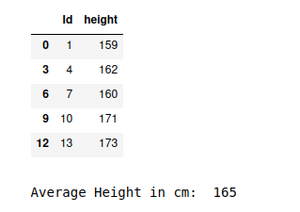## Types of Systematic Sampling

Systematic Sampling is of three types as depicted below :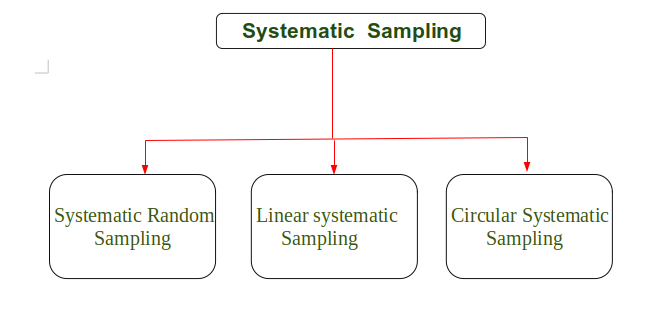Types of Systematic Sampling

### Systematic Random Sampling:

In systematic random Sampling, random starting point is chosen and after that from that random starting point systematic sampling is applied.

Approach:

• Get data
• Choose a random starting point
• Apply systematic approach to the data
• Perform operations as intended

Example:

## Python3

 `# Import in order to use inbuilt functions``import` `numpy as np``import` `pandas as pd``import` `random`` ` `# Define total number of house``number_of_house ``=` `30`` ` `# Create data dictionary``data ``=` `{``'house_number'``: [``1``, ``2``, ``3``, ``4``, ``5``, ``6``, ``7``, ``8``, ``9``, ``10``, ``11``, ``12``, ``13``,``                         ``14``, ``15``, ``16``, ``17``, ``18``, ``19``, ``20``, ``21``, ``22``, ``23``,``                         ``24``, ``25``, ``26``, ``27``, ``28``, ``29``, ``30``],``        ``'number_of_children'``: [``2``, ``2``, ``1``, ``3``, ``2``, ``1``, ``4``, ``1``, ``3``, ``5``, ``4``, ``3``, ``5``,``                               ``3``, ``2``, ``1``, ``2``, ``3``, ``4``, ``5``, ``3``, ``4``, ``5``, ``2``, ``2``, ``2``,``                               ``2``, ``3``, ``2``, ``1``]}`` ` `# Transform dictionary into a data frame``df ``=` `pd.DataFrame(data)`` ` `# Defining Size of Systematic Sample``size_of_systematic_sample ``=` `6`` ` `# Defining Interval(gap) in order to get required data.``interval ``=` `(number_of_house ``/``/` `size_of_systematic_sample)`` ` `# Choosing Random Numner``random_number ``=` `random.randint(``1``, ``30``)`` ` `# Define systematic sampling function``def` `systematic_sampling(df, step):`` ` `    ``indexes ``=` `np.arange(random_number, ``len``(df), step``=``step)``    ``systematic_sample ``=` `df.iloc[indexes]``    ``return` `systematic_sample`` ` ` ` `# Obtain a systematic sample and save it in a new variable``systematic_sample ``=` `systematic_sampling(df, interval)`` ` `# View sampled data frame``display(systematic_sample)`` ` `# Empty Print Statement for new line``print``()`` ` `# Save the sample data in a separate variable``systematic_data ``=` `round``(systematic_sample[``'number_of_children'``].mean())`` ` `# Printig Avergae Number of Children``print``(``"Average Number Of Childrens in Locality: "``, systematic_data)`

Output: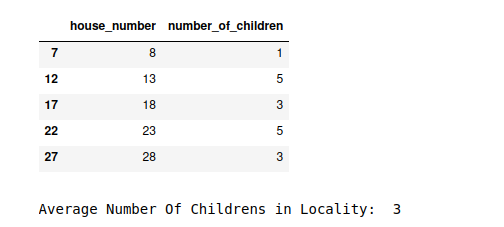### Linear Systematic Sampling :

Linear Systematic Sampling is a type of systematic sampling where the sample are selected using linear approach. Linear approach in the sense that after particular interval the sample is selected from the large data and after that operations are performed on the Selected Sample.

The elements are chosen between the range starting_random_number to last_element -1.

Approach:

• Get data
• Select data from the dataset after a particular interval
• Perform operations as intended

Example:

## Python3

 `# Import in order to use inbuilt functions``import` `numpy as np``import` `pandas as pd``import` `random`` ` `# Define total number of boxes``number_of_boxes ``=` `30`` ` `# Create data dictionary``data ``=` `{``'Box_Number'``: [``1``, ``2``, ``3``, ``4``, ``5``, ``6``, ``7``, ``8``, ``9``, ``10``, ``11``, ``12``, ``13``, ``14``, ``                       ``15``, ``16``, ``17``, ``18``, ``19``, ``20``, ``21``, ``22``, ``23``, ``24``, ``25``, ``26``,``                       ``27``, ``28``, ``29``, ``30``],``         ` `        ``'Defective_Bulbs'``: [``2``, ``2``, ``1``, ``0``, ``2``, ``1``, ``0``, ``1``, ``3``, ``5``, ``4``, ``3``, ``5``, ``3``, ``                            ``0``, ``1``, ``2``, ``0``, ``4``, ``5``, ``3``, ``4``, ``5``, ``2``, ``0``, ``3``, ``2``, ``0``,``                            ``5``, ``4``]}`` ` `# Transform dictionary into a data frame``df ``=` `pd.DataFrame(data)`` ` ` ` `# Size of Systematic Sample``size_systematic_sample ``=` `5`` ` `# Interval (Gap) taken``interval ``=` `(number_of_boxes ``/``/` `size_systematic_sample)`` ` `# Choosing Random Starting Point``random_number ``=` `random.randint(``1``, ``30``)`` ` `# Define systematic sampling function``def` `systematic_sampling(df, step):`` ` `    ``indexes ``=` `np.arange(random_number, ``len``(df)``-``1``, step``=``step)``    ``systematic_sample ``=` `df.iloc[indexes]``    ``return` `systematic_sample`` ` ` ` `# Obtain a systematic sample and save it in a new variable``systematic_sample ``=` `systematic_sampling(df, interval)`` ` `# View sampled data frame``display(systematic_sample)`` ` `# Empty Print Statement for new line``print``()`` ` `# Save the sample data in a separate variable``systematic_data ``=` `round``(systematic_sample[``'Defective_Bulbs'``].mean())`` ` `# Printig Avergae Number of Defective Bulbs``print``(``"Average Number Of Defective Bulbs: "``, systematic_data)`

Output: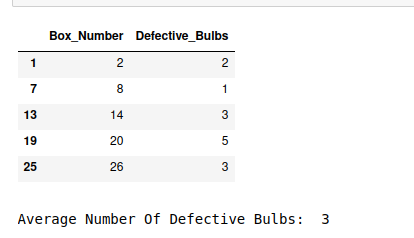### Circular Systematic Sampling

In Circular Systematic Sampling, a sample again starts from the same point after ending. Basically, while selecting samples systematically and when ending element is reached, once again the selecting of sample will start from the beginning until all the elements of sample are selected. It means operations are performed on all the data which is selected using Circular Systematic Sampling.

Approach:

• Get data
• Select samples systematically
• Once end is reached, restart
• Perform operations as intended

Program:

## Python3

 `# Import in order to use inbuilt functions``import` `numpy as np``import` `pandas as pd``import` `random`` ` `# Define total number of house``number_of_house ``=` `30`` ` `# Create data dictionary``data ``=` `{``'house_number'``: [``1``, ``2``, ``3``, ``4``, ``5``, ``6``, ``7``, ``8``, ``9``, ``10``, ``11``, ``12``, ``13``, ``                         ``14``, ``15``, ``16``, ``17``, ``18``, ``19``, ``20``, ``21``, ``22``, ``23``,``                         ``24``, ``25``, ``26``, ``27``, ``28``, ``29``, ``30``],``        ``'number_of_Adults'``: [``2``, ``2``, ``5``, ``3``, ``2``, ``8``, ``4``, ``7``, ``8``, ``5``, ``4``, ``9``, ``5``,``                             ``4``, ``2``, ``3``, ``2``, ``3``, ``4``, ``5``, ``6``, ``4``, ``5``, ``4``, ``2``, ``6``,``                             ``2``, ``3``, ``2``, ``2``]}`` ` `# Transform dictionary into a data frame``df ``=` `pd.DataFrame(data)`` ` `# Defining Size of Systematic Sample``size_of_systematic_sample ``=` `6`` ` ` ` `# Defining Interval(gap) in order to get required data.``interval ``=` `(number_of_house ``/``/` `size_of_systematic_sample)`` ` `# Define systematic sampling function``def` `systematic_sampling(df, step):``    ``indexes ``=` `np.arange(``0``, ``len``(df), step``=``step)``    ``systematic_sample ``=` `df.iloc[indexes]``    ``return` `systematic_sample`` ` ` ` `# Obtain a systematic sample and save it in a new variable``systematic_sample ``=` `systematic_sampling(df, interval)`` ` `# View sampled data frame``display(systematic_sample)`` ` ` ` `# Empty Print Statement for new line``print``()`` ` `# Save the sample data in a separate variable``systematic_data ``=` `round``(systematic_sample[``'number_of_Adults'``].mean())`` ` `# Printig Avergae Number of Children``print``(``"Average Number Of Adults in Locality: "``, systematic_data)`

Output: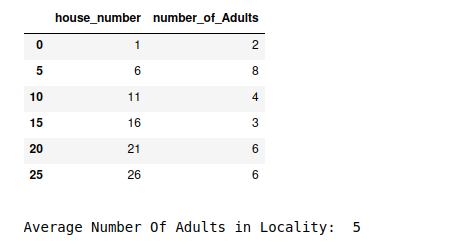Attention geek! Strengthen your foundations with the Python Programming Foundation Course and learn the basics.

To begin with, your interview preparations Enhance your Data Structures concepts with the Python DS Course.

My Personal Notes arrow_drop_up